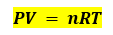# Problem: A spray can is used until it is empty except for the propellant gas, which has a pressure of 1344 torr at 23 °C. If the can is thrown into a fire (T = 475 °C), what will be the pressure in the hot can?

###### FREE Expert Solution

Recall the ideal gas equation:Assuming that the volume of the spray can and the amount of the gas will remain constant, and R is also a constant.

91% (77 ratings)###### Problem Details

A spray can is used until it is empty except for the propellant gas, which has a pressure of 1344 torr at 23 °C. If the can is thrown into a fire (T = 475 °C), what will be the pressure in the hot can?